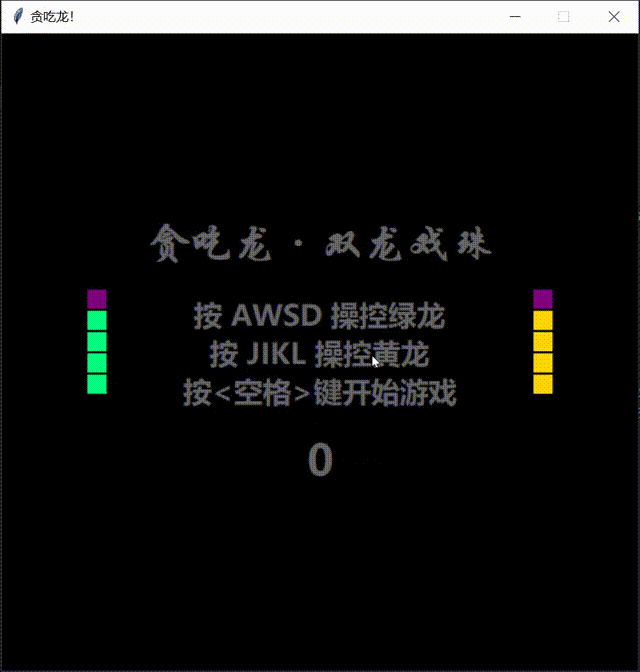> 文档中心 > 摸鱼宝典（一）——贪吃蛇游戏改版：贪吃龙 · 双龙戏珠小游戏（Python）

# 摸鱼宝典（一）——贪吃蛇游戏改版：贪吃龙 · 双龙戏珠小游戏（Python）

啊哈哈哈，最近闲来无事，随手写了个小游戏，在学习的时候摸鱼和划水那是必备啊！还等什么？快来体验这款独特的改版贪吃蛇 ——《贪吃龙 · 双龙戏珠》小游戏吧！

Tip：源码下载链接在文章最下面

## 【游戏效果】## 【教程环节】

【完整源码在后面，可以直接参考】

### 步骤一：引入模块

``from tkinter import *# 引入界面化编程模块from random import *# 引入随机数模块``

### 步骤二：设置全局变量

``Food,Score,Life = [None,None],0,1# 设置初始食物位置、初始分数、是否存活（1==True 0==False）``

### 步骤三：Main主类初始化

``class Main:    '''    ### 主类    ---    类似于C语言中main函数    用类实现只是为了方便，在这个程序中没其他作用    '''    def __init__(self): ''' 界面的初始化 ''' self.game = Tk()# 初始化界面 self.game.title('贪吃龙！')# 界面标题 self.game.geometry('600x600+300+50') #界面大小及位置（600×600像素，偏移屏幕左上角横向300像素，纵向50像素） self.game.resizable(0,0)# 窗口横向、纵向大小是否可以拉伸（0==False 1==True） self.canvas = Canvas(self.game,bg='black',highlightthickness=0)# 初始化画布控件，背景黑色，高亮边框厚度为0 self.canvas.place(width=600,height=600)# 画布控件左上角置于(0,0)[默认值] 宽600像素、高600像素 self.canvas.create_text(300,200,text='贪吃龙·双龙戏珠',font=('华文行楷',30,'bold'),fill='#666666')# 字样1 self.canvas.create_text(300,300,text='按 AWSD 操控绿龙\n按 JIKL 操控黄龙\n按键开始游戏',font=('微软雅黑',20,'bold'),fill='#666666',justify='center')# 字样2 self.score = self.canvas.create_text(300,400,text='0',font=('微软雅黑',30,'bold'),fill='#666666')# 得分字样 self.s1 = Snake(self.game,self.canvas,4,12,'springgreen')# 实例化第一条 Snake(类) self.s2 = Snake(self.game,self.canvas,25,12,'gold')# 实例化第二条 Snake(类) 【有兴趣的可以实例化第N条】 self.game.bind('',self.start)# 空格键键盘关联 start 方法 self.game.mainloop()# 消息事件循环``

### 步骤四：编写Main类的start方法、move方法和fresh方法

``    def start(self,event:Event):# event 参数没有用到但不能删去，为了与前面形成关联 ''' 游戏启动 ''' self.game.unbind('')# 取消空格键的关联 self.move()# 键盘关联开始有反应 self.fresh()# 游戏画面开始更新``

``    def move(self): ''' 键盘关联 ''' self.game.bind('',self.s1.move)# a 按键检测 self.game.bind('',self.s1.move)# w 按键检测 self.game.bind('',self.s1.move)# s 按键检测 self.game.bind('',self.s1.move)# d 按键检测 self.game.bind('',self.s2.move)# j 按键检测 self.game.bind('',self.s2.move)# i 按键检测 self.game.bind('',self.s2.move)# k 按键检测 self.game.bind('',self.s2.move)# l 按键检测``

``    def fresh(self): ''' 画面更新 ''' global Food,Score,Life# 声明为全局变量，方便对它们的修改 if Food == [None,None]:# 判断界面上是不是没有食物，没有才会刷新食物     x,y = randint(0,29),randint(0,29)# 随机产生食物的坐标 (x*20,y*20)     Food = [self.canvas.create_rectangle(20*x+1,20*y+1,20*(x+1)-1,20*(y+1)-1,fill='red',width=0),[x,y]]# 产生食物 self.s1.update()# 更新 s1 的位置 self.s2.update()# 更新 s2 的位置 self.canvas.itemconfigure(self.score,text=str(Score))# 更新分数字样 if Life:self.game.after(int(100-Score**0.5),self.fresh)# 如果 Life 不为 0(False) 就继续“更新”``

### 步骤五：Snake蛇类的初始化

``class Snake:    '''    ### 蛇类     ---    实例化一个就可以实现一个单独的蛇    Example:    `s1 = Snake(self.game,self.canvas,4,12,'springgreen')`    就可以实现一个颜色为'springgreen'，头部左上角位置在(4×20,12*20)的“蛇”    '''    def __init__(self,tk:Tk,canvas:Canvas,x:int,y:int,color:str): self.game = tk self.canvas = canvas i1 = self.canvas.create_rectangle(20*x+1,20*y+1,20*(x+1)-1,20*(y+1)-1,width=0,fill='purple')# 初始化蛇头 i2 = self.canvas.create_rectangle(20*x+1,20*(y+1)+1,20*(x+1)-1,20*(y+2)-1,width=0,fill=color)# 初始化蛇身1 i3 = self.canvas.create_rectangle(20*x+1,20*(y+2)+1,20*(x+1)-1,20*(y+3)-1,width=0,fill=color)# 初始化蛇身2 i4 = self.canvas.create_rectangle(20*x+1,20*(y+3)+1,20*(x+1)-1,20*(y+4)-1,width=0,fill=color)# 初始化蛇身3 i5 = self.canvas.create_rectangle(20*x+1,20*(y+4)+1,20*(x+1)-1,20*(y+5)-1,width=0,fill=color)# 初始化蛇身4 self.snake = [[i1,'w'],[i2,'w'],[i3,'w'],[i4,'w'],[i5,'w']]# 将蛇的数据放入蛇数据列表方便分析 self.head,self.tail,self.color = [x,y],[x,y+4],color# 记录当前蛇头位置、当前蛇尾位置、蛇身颜色``

### 步骤六：Snake类的move方法和update方法

``    def move(self,event:Event): ''' 移动的区分 ''' if event.char in ['a','j']:self.snake = 'a'# 按 a 或 j 键向左 elif event.char in ['w','i']:self.snake = 'w'# 按 w 或 i 键向上 elif event.char in ['s','k']:self.snake = 's'# 按 s 或 k 键向下 elif event.char in ['d','l']:self.snake = 'd'# 按 d 或 l 键向右``

event.char 代表键盘输入的字符。

``    def update(self): ''' 更新蛇的位置 ''' for k in range(len(self.snake)-1,-1,-1):# 遍历蛇数据列表(必须倒遍历！)     dx = 0 if self.snake[k] in ['w','s'] else 20 if self.snake[k] == 'd' else -20# 解析当前蛇身在水平方向应该的位移     dy = 0 if self.snake[k] in ['a','d'] else 20 if self.snake[k] == 's' else -20# 解析当前蛇身在垂直方向应该的位移     self.canvas.move(self.snake[k],dx,dy)# 更新当前蛇身位置     if k:self.snake[k] = self.snake[k-1]# 更新当前蛇身下次前行的方向（就是更新为前一个的蛇身的方向）     else:# else的情况只能是 k 为 0，也就是蛇头位置  hx,hy = self.head# 读取旧蛇头位置  self.head = [hx+dx//20,hy+dy//20]# 更新蛇头位置数据     if k == len(self.snake)-1:# 判断是否为蛇尾  tx,ty = self.tail# 读取旧蛇尾位置  self.tail = [tx+dx//20,ty+dy//20]# 更新蛇尾位置数据 self.dead()# 判定是否撞墙死亡 self.eat()# 判定是否吃掉食物``

``    def dead(self): ''' 检测是否撞墙死亡，实则是坐标位置越界检测 ''' global Life# 声明全局变量，方便对其的修改 x,y = self.head# 蛇头的位置 if not (0<=x<=29 and 0<=y<=29):# 检测蛇头位置是否越界（超出屏幕）     Life = 0# Life 设为 0(False) 标识死亡     self.canvas.create_text(300,250,text='You\nDead',fill='red',font=('华文新魏',100,'bold'),justify='center')# 产生死亡字样``

``    def eat(self): ''' 检测是否吃掉食物，有点类似于碰撞检测(但又没有碰撞检测那么复杂) ''' global Food,Score# 声明全局变量，方便对其的修改 if self.head == Food:# 当前蛇头位置与食物位置重合，判定为吃掉食物     self.canvas.delete(Food)# 删去食物的图像     Food = [None,None]# 设置食物坐标为空，即屏幕中没有食物了     Score += 10# 得分加10     x,y = self.tail# 读取当前蛇尾位置，准备给蛇增加长度     dx = 0 if self.snake[-1] in ['w','s'] else 20 if self.snake[-1] == 'd' else -20# 判断水平方向上蛇的位移     dy = 0 if self.snake[-1] in ['a','d'] else 20 if self.snake[-1] == 's' else -20# 判断垂直方向上蛇的位移     x -= dx//20;y -= dy//20# 解析新蛇尾应该的实际坐标     self.tail = [x,y]# 更新蛇尾坐标为新的蛇尾坐标     self.snake.append([self.canvas.create_rectangle(20*x+1,20*y+1,20*(x+1)-1,20*(y+1)-1,width=0,fill=self.color),self.snake[-1]])# 蛇数据列表加上新蛇尾的数据``

### 步骤八：实例化Main类，开始游戏

``Main()``

## 【完整源码】

``from tkinter import *# 引入界面化编程模块from random import *# 引入随机数模块Food,Score,Life = [None,None],0,1# 设置初始食物位置、初始分数、是否存活（1==True 0==False）class Snake:    '''    ### 蛇类     ---    实例化一个就可以实现一个单独的蛇    Example:    `s1 = Snake(self.game,self.canvas,4,12,'springgreen')`    就可以实现一个颜色为'springgreen'，头部左上角位置在(4×20,12*20)的“蛇”    '''    def __init__(self,tk:Tk,canvas:Canvas,x:int,y:int,color:str): self.game = tk self.canvas = canvas i1 = self.canvas.create_rectangle(20*x+1,20*y+1,20*(x+1)-1,20*(y+1)-1,width=0,fill='purple')# 初始化蛇头 i2 = self.canvas.create_rectangle(20*x+1,20*(y+1)+1,20*(x+1)-1,20*(y+2)-1,width=0,fill=color)# 初始化蛇身1 i3 = self.canvas.create_rectangle(20*x+1,20*(y+2)+1,20*(x+1)-1,20*(y+3)-1,width=0,fill=color)# 初始化蛇身2 i4 = self.canvas.create_rectangle(20*x+1,20*(y+3)+1,20*(x+1)-1,20*(y+4)-1,width=0,fill=color)# 初始化蛇身3 i5 = self.canvas.create_rectangle(20*x+1,20*(y+4)+1,20*(x+1)-1,20*(y+5)-1,width=0,fill=color)# 初始化蛇身4 self.snake = [[i1,'w'],[i2,'w'],[i3,'w'],[i4,'w'],[i5,'w']]# 将蛇的数据放入蛇数据列表方便分析 self.head,self.tail,self.color = [x,y],[x,y+4],color# 记录当前蛇头位置、当前蛇尾位置、蛇身颜色    def eat(self): ''' 检测是否吃掉食物，有点类似于碰撞检测(但又没有碰撞检测那么复杂) ''' global Food,Score# 声明全局变量，方便对其的修改 if self.head == Food:# 当前蛇头位置与食物位置重合，判定为吃掉食物     self.canvas.delete(Food)# 删去食物的图像     Food = [None,None]# 设置食物坐标为空，即屏幕中没有食物了     Score += 10# 得分加10     x,y = self.tail# 读取当前蛇尾位置，准备给蛇增加长度     dx = 0 if self.snake[-1] in ['w','s'] else 20 if self.snake[-1] == 'd' else -20# 判断水平方向上蛇的位移     dy = 0 if self.snake[-1] in ['a','d'] else 20 if self.snake[-1] == 's' else -20# 判断垂直方向上蛇的位移     x -= dx//20;y -= dy//20# 解析新蛇尾应该的实际坐标     self.tail = [x,y]# 更新蛇尾坐标为新的蛇尾坐标     self.snake.append([self.canvas.create_rectangle(20*x+1,20*y+1,20*(x+1)-1,20*(y+1)-1,width=0,fill=self.color),self.snake[-1]])# 蛇数据列表加上新蛇尾的数据    def dead(self): ''' 检测是否撞墙死亡，实则是坐标位置越界检测 ''' global Life# 声明全局变量，方便对其的修改 x,y = self.head# 蛇头的位置 if not (0<=x<=29 and 0<=y<=29):# 检测蛇头位置是否越界（超出屏幕）     Life = 0# Life 设为 0(False) 标识死亡     self.canvas.create_text(300,250,text='You\nDead',fill='red',font=('华文新魏',100,'bold'),justify='center')# 产生死亡字样    def update(self): ''' 更新蛇的位置 ''' for k in range(len(self.snake)-1,-1,-1):# 遍历蛇数据列表(必须倒遍历！)     dx = 0 if self.snake[k] in ['w','s'] else 20 if self.snake[k] == 'd' else -20# 解析当前蛇身在水平方向应该的位移     dy = 0 if self.snake[k] in ['a','d'] else 20 if self.snake[k] == 's' else -20# 解析当前蛇身在垂直方向应该的位移     self.canvas.move(self.snake[k],dx,dy)# 更新当前蛇身位置     if k:self.snake[k] = self.snake[k-1]# 更新当前蛇身下次前行的方向（就是更新为前一个的蛇身的方向）     else:# else的情况只能是 k 为 0，也就是蛇头位置  hx,hy = self.head# 读取旧蛇头位置  self.head = [hx+dx//20,hy+dy//20]# 更新蛇头位置数据     if k == len(self.snake)-1:# 判断是否为蛇尾  tx,ty = self.tail# 读取旧蛇尾位置  self.tail = [tx+dx//20,ty+dy//20]# 更新蛇尾位置数据 self.dead()# 判定是否撞墙死亡 self.eat()# 判定是否吃掉食物    def move(self,event:Event): ''' 移动的区分 ''' if event.char in ['a','j']:self.snake = 'a'# 按 a 或 j 键向左 elif event.char in ['w','i']:self.snake = 'w'# 按 w 或 i 键向上 elif event.char in ['s','k']:self.snake = 's'# 按 s 或 k 键向下 elif event.char in ['d','l']:self.snake = 'd'# 按 d 或 l 键向右class Main:    '''    ### 主类    ---    类似于C语言中main函数    用类实现只是为了方便，在这个程序中没其他作用    '''    def __init__(self): ''' 界面的初始化 ''' self.game = Tk()# 初始化界面 self.game.title('贪吃龙！')# 界面标题 self.game.geometry('600x600+300+50') #界面大小及位置（600×600像素，偏移屏幕左上角横向300像素，纵向50像素） self.game.resizable(0,0)# 窗口横向、纵向大小是否可以拉伸（0==False 1==True） self.canvas = Canvas(self.game,bg='black',highlightthickness=0)# 初始化画布控件，背景黑色，高亮边框厚度为0 self.canvas.place(width=600,height=600)# 画布控件左上角置于(0,0)[默认值] 宽600像素、高600像素 self.canvas.create_text(300,200,text='贪吃龙·双龙戏珠',font=('华文行楷',30,'bold'),fill='#666666')# 字样1 self.canvas.create_text(300,300,text='按 AWSD 操控绿龙\n按 JIKL 操控黄龙\n按键开始游戏',font=('微软雅黑',20,'bold'),fill='#666666',justify='center')# 字样2 self.score = self.canvas.create_text(300,400,text='0',font=('微软雅黑',30,'bold'),fill='#666666')# 得分字样 self.s1 = Snake(self.game,self.canvas,4,12,'springgreen')# 实例化第一条 Snake(类) self.s2 = Snake(self.game,self.canvas,25,12,'gold')# 实例化第二条 Snake(类) 【有兴趣的可以实例化第N条】 self.game.bind('',self.start)# 空格键键盘关联 start 方法 self.game.mainloop()# 消息事件循环    def start(self,event:Event):# event 参数没有用到但不能删去，为了与前面形成关联 ''' 游戏启动 ''' self.game.unbind('')# 取消空格键的关联 self.move()# 键盘关联开始有反应 self.fresh()# 游戏画面开始更新    def fresh(self): ''' 画面更新 ''' global Food,Score,Life# 声明为全局变量，方便对它们的修改 if Food == [None,None]:# 判断界面上是不是没有食物，没有才会刷新食物     x,y = randint(0,29),randint(0,29)# 随机产生食物的坐标 (x*20,y*20)     Food = [self.canvas.create_rectangle(20*x+1,20*y+1,20*(x+1)-1,20*(y+1)-1,fill='red',width=0),[x,y]]# 产生食物 self.s1.update()# 更新 s1 的位置 self.s2.update()# 更新 s2 的位置 self.canvas.itemconfigure(self.score,text=str(Score))# 更新分数字样 if Life:self.game.after(int(100-Score**0.5),self.fresh)# 如果 Life 不为 0(False) 就继续“更新”    def move(self): ''' 键盘关联 ''' self.game.bind('',self.s1.move)# a 按键检测 self.game.bind('',self.s1.move)# w 按键检测 self.game.bind('',self.s1.move)# s 按键检测 self.game.bind('',self.s1.move)# d 按键检测 self.game.bind('',self.s2.move)# j 按键检测 self.game.bind('',self.s2.move)# i 按键检测 self.game.bind('',self.s2.move)# k 按键检测 self.game.bind('',self.s2.move)# l 按键检测Main()# 类似于程序的入口``

【下载链接】